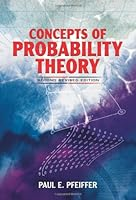# Concepts of Probability Theory: 2nd Edition## Book Description

Concepts of Probability : Second Revised Edition (Dover Books on )

Using the simple conceptual of the Kolmogorov model, this intermediate-level textbook discusses random variables and probability distributions, sums and integrals, expectation, sequence and sums of random variables, and random processes. For advanced undergraduate students of science, engineering, or mathematics acquainted with basic calculus. Includes problems with answers and six appendixes. 1965 edition.

Chapter 1. Introduction
Chapter 2 A mathematical model for probability
Chapter 3. Random Variables and Probability Distributions
Chapter 4. Sums and Integrals
Chapter 5. Mathematical Expectation
Chapter 6. Sequences and Sums of Random Variables
Chapter 7. Random Processes
Appendix A. Some Elements of Combinatorial
Appendix B. Some Topics in Set Theory
Appendix C. Measurability of
Appendix D. Proofs of Some Theorems
Appendix E. Integrals of Complex-valued Random Variables
Appendix F. Summary of Properties and Key Theorems
bibliography
Index

## Book Details

• Title: Concepts of Probability Theory: 2nd Edition
• Author:
• Length: 416 pages
• Edition: 2
• Language: English
• Publisher:
• Publication Date: 2012-06-13
• ISBN-10: 0486636771
• ISBN-13: 9780486636771